# Running total sums the current value and all those that precede it. Running totals are used to update totals within a series in MS Excel. For Example, you may use running total to calculate account balance, number of students, cumulative sales ets.

Running total changes each time new data is Added, Removed or Edited to a list.

# How to Create Running Total (Cumulative Sum) in MS Excel

Let's learn with Example
>> Select cell B15 , Enter SUM function
B15 equals =SUM(B3:B14)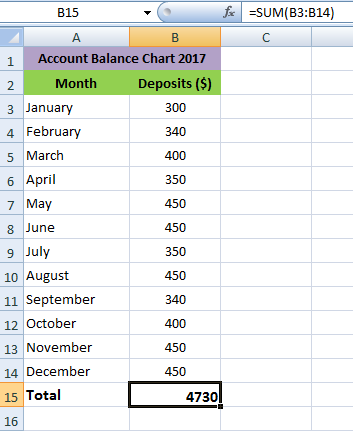>> Select cell C3 and enter the SUM function
C3 equals =SUM(B\$3:B3)
** (B\$3) is a mixed reference. We fixed the reference to row 3 by adding a \$ symbol in front of the row number. (B3) is a normal relative reference.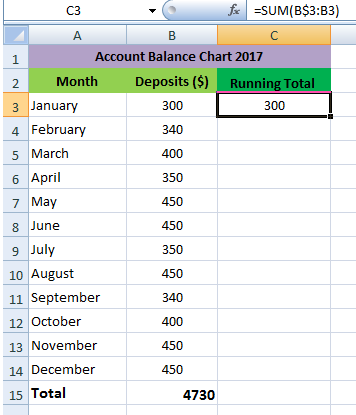>> Select cell C3, click on the lower right corner and drag it down to cell C14, the Formula will be copied To all the cells.
** When we drag the formula down, the mixed reference (B\$3) remains same, but the relative reference (B3) changes to B4, B5, B6 and so on.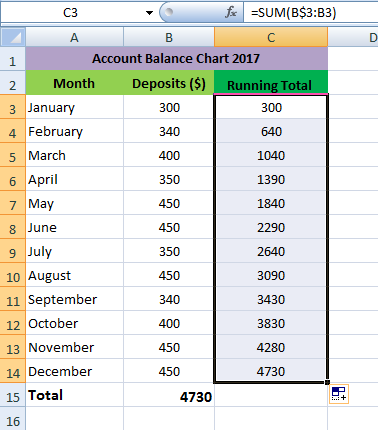Let's Check
>> Select cell C4 and look at the formula.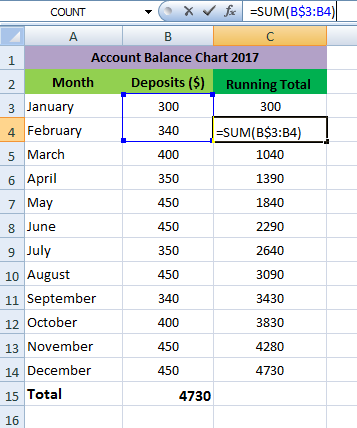>> Select cell C5 and look at the formula.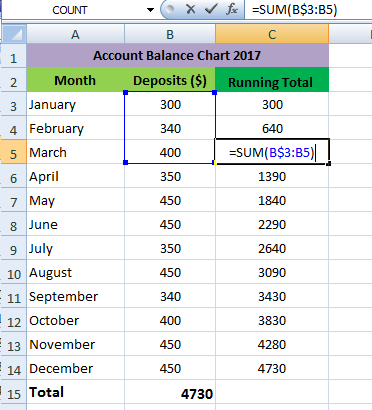Likewise, All the cells have Formula.
Running total changes each time new data is Added, Removed or Edited to a list.
Let's Learn with Example
>> Edit the data in December Month.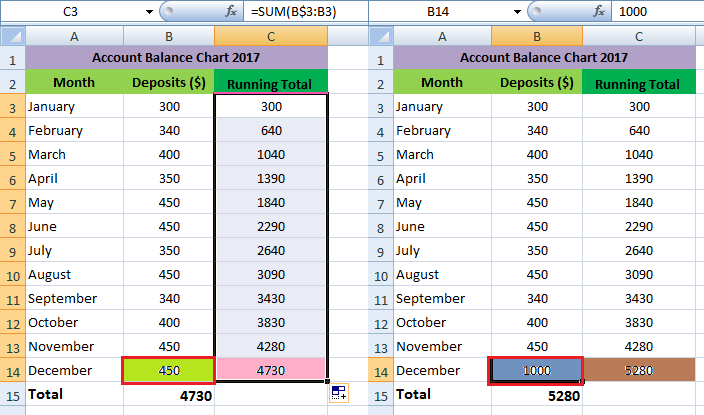It can be seen , when data is edited the Running total also changed.